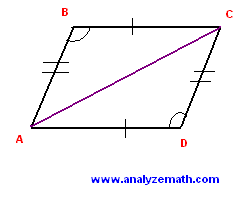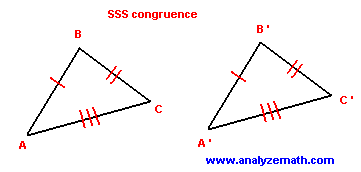# Congruent Triangles Problems with Solutions

Postulates and theorems on congruent triangles are discussed using examples. More problems on congruent triangles with detailed solutions are included.

## Side-Angle-Side (SAS) Congruence Postulate

If two sides (CA and CB) and the included angle ( BCA ) of a triangle are congruent to the corresponding two sides (C'A' and C'B') and the included angle (B'C'A') in another triangle, then the two triangles are congruent.### Example 1

Let ABCD be a parallelogram and AC be one of its diagonals. What can you say about triangles ABC and CDA? Explain your answer.Solution to Example 1

• In a parallelogram, opposite sides are congruent. Hence sides
BC and AD are congruent, and also sides AB and CD are congruent.
• In a parallelogram opposite angles are congruent. Hence angles
ABC and CDA are congruent.
• Two sides and an included angle of triangle ABC are congruent to two corresponding sides and an included angle in triangle CDA. According to the above postulate the two triangles ABC and CDA are congruent.

## Side-Side-Side (SSS) Congruence Postulate

If the three sides (AB, BC and CA) of a triangle are congruent to the corresponding three sides (A'B', B'C' and C'A') in another triangle, then the two triangles are congruent.### Example 2

Let ABCD be a square and AC be one of its diagonals. What can you say about triangles ABC and CDA? Explain your answer.Solution to Example 2

• In a square, all four sides are congruent. Hence sides
AB and CD are congruent, and also sides BC and DA are congruent.
• The two triangles also have a common side: AC. Triangles ABC has three sides congruent to the corresponding three sides in triangle CDA. According to the above postulate the two triangles are congruent. The triangles are also right triangles and isosceles.

## Angle-Side-Angle (ASA) Congruence Postulate

If two angles (ACB, ABC) and the included side (BC) of a triangle are congruent to the corresponding two angles (A'C'B', A'B'C') and included side (B'C') in another triangle, then the two triangles are congruent.### Example 3

ABC is an isosceles triangle. BB' is the angle bisector. Show that triangles ABB' and CBB' are congruent.Solution to Example 3

• Since ABC is an isosceles triangle its sides AB and BC are congruent and also its angles BAB' and BCB' are congruent. Since BB' is an angle bisector, angles ABB' and CBB' are congruent.
Two angles and an included side in triangles ABB' are congruent to two corresponding angles and one included side in triangle CBB'. According to the above postulate triangles ABB' and CBB' are congruent.

## Angle-Angle-Side (AAS) Congruence Theorem

If two angles (BAC, ACB) and a side opposite one of these two angles (AB) of a triangle are congruent to the corresponding two angles (B'A'C', A'C'B') and side (A'B') in another triangle, then the two triangles are congruent.### Example 4Solution to Example 4

• In triangle ABC, the third angle ABC may be calculated using the theorem that the sum of all three angles in a triangle is equal to 180 derees. Hence
angle ABC = 180 - (25 + 125) = 30 degrees
• The two triangles have two congruent corresponding angles and one congruent side.
angles ABC and QPR are congruent. Also angles BAC and PQR are congruent. Sides BC and PR are congruent.
• Two angles and one side in triangle ABC are congruent to two corresponding angles and one side in triangle PQR. According to the above theorem they are congruent.

## Right Triangle Congruence Theorem

If the hypotenuse (BC) and a leg (BA) of a right triangle are congruent to the corresponding hypotenuse (B'C') and leg (B'A') in another right triangle, then the two triangles are congruent.### Example 5

Show that the two right triangles shown below are congruent.Solution to Example 5

• We first use Pythagora's theorem to find the length of side AB in triangle ABC.
length of AB = sqrt [5 2 - 3 2] = 4
• One leg and the hypotenuse in triangle ABC are congruent to a corresponding leg and hypotenuse in the right triangle A'B'C'. According to the above theorem, triangles ABC and B'A'C' are congruent.

## Problems with Detailed Solutions

### Problem 1

In the isosceles triangle ABC, BA and BC are congruent. M and N are points on AC such that MA is congruent to MB and NB is congruent to NC. Show that triangles AMB and CNB are congruent.Solution to Problem 1:

• Since triangle ABC is isosceles and BA and BC are congruent then angles BAM and BCN are congruent.
• Also since MA is congruent to MB, then AMB is an isosceles triangle and angles BAM and ABM are congruent. NB and NC are also congruent; CNB is an isosceles triangle and angles CBN and BCN are congruent. In fact all four angles BAM, ABM, CBN and BCN are congruent. Comparing triangles BAM and CNB, they have corresponding sides AB and BC congruent, corresponding angles BAM and BCN congruent and corresponding angles ABM and CBN congruent. These two triangles are therefore congruent. This is the ASA congruent case.

### Problem 2

ABCD is a parallelogram and BEFC is a square. Show that triangles ABE and DCF are congruent.Solution to Problem 2:

• In the parallelogram ABCD, BA is congruent to CD. In the square BEFC, EB is congruent to FC. Since EB is parallel to FC and BA is parallel to CD then angles EBA and FCD are congruent. Comparing triangles ABE and DCF: angle EBA included between EB and BA in triangle ABE is congruent to angles FCD included between sides FC and CD. EB is congruent to FC and BA is congruent to CD. These two triangles are congruent. It is the SAS congruent case.

### Problem 3

ABCD is a square. C' is a point on BA and B' is a point on AD such that BB' and CC'are perpendicular. Show that AB'B and BC'C are congruent.Solution to Problem 3:
• Since ABCD is a square angles CBC' and BAB' are right angles and therefore congruent. Also side BA is congruent to side BC. BC and AD are parallel and BB' is a transverse, therefore angles OBC and BB'A are interior alternate angles and are congruent.
• Since CC' and BB' are perpendicular, then triangle CBO is rectangle at point O and therefore
size of angle OBC + size of angle BCO = 90 degrees
• ABB' is also a right triangle and therefore
size of angle ABB' + size of angle BB'A = 90 degrees
• Combine the above equations with the fact that angles OBC and BB'A are congruent, we can conclude that
size of angle ABB' = size of angle BCC'
• Triangles AB'B and BC'C have side BC congruent to side BA; angle BCC' congruent to angle ABB' and angle BAB' congruent to angle CBC' are congruent. The two triangles are congruent. This is the ASA congruent case.

### Problem 4

ABC is a triangle and M is the midpoint of AC. I and J are points on BM such that AI and CJ are perpendicular to BM. Show that triangles AIM and CJM are congruent.Solution to Problem 4:
• Since M is the midpoint of AC then AM is congruent to MC. AI and CJ are perpendicular to the same line BM and are therefore parallel with CA as the transverse. Angles MAI and MCJ are interior alternate angles and therefore congruent. Angles AMI and JMC are vertical angles and therefore congruent. Triangles AIM and CJM have one congruent side between two congruent angles and are therefore congruent. This is the ASA congruent case.

### Problem 5

ABC is an isosceles triangle with BA and BC congruent. Point K is on AB and point L is on BC. Both KK' and LL' are perpendicular to AC. Segments KK' and LL' are congruent. Show that KK'M and LL'M are congruent.Solution to Problem 5:

• KK' and LL' are perpendicular to the same line AC and are therefore parallel to each other. KL is transverse to these two lines and K'KM and L'LM are interior alternate angles and therefore congruent. Angles KK'M and LL'M are congruent since the size of each is 90 degrees. The two triangles KK'M and LL'M have one corresponding congruent side between two congruent angles and are therefor congruent.

## More References and Links to Geometry Tutorials

Similar Triangles Examples and Problems with Solutions
Triangles
Properties of Triangles
Geometry Tutorials